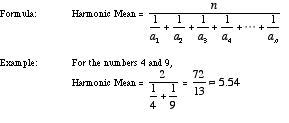# harmonic mean

Harmonic Mean

A kind of average. To find the harmonic mean of a set of n numbers, add the reciprocals of the numbers in the set, divide the sum by n, then take the reciprocal of the result. The harmonic mean of {a1, a2, a3, a4, . . ., an} is given below.